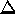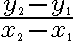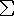# Linear Regression

#### Lesson Overview

Last lesson we introduced correlation and the correlation coefficients of Pearson and Spearman. In this lesson we come up with linear regression equations.

### Linear Regression

Regression goes one step beyond correlation in identifying the relationship between two variables. It creates an equation so that values can be predicted within the range framed by the data. This is known as interpolation. To go beyond the observations is fraught with peril and is known as extrapolation. However, doing so to determine the federal deficit or necessary pension funding levels are nonetheless important applications.

Since the discussion is on linear correlations and the predicted values need to be as close as possible to the data, the equation is called the best-fitting line or regression line. The regression line was named after the work Galton did in gene characteristics that reverted (regressed) back to a mean value. That is, tall parents had children closer to the average.

Slope is an important concept so we will review some important facts here.

 slope = m = rise/run = dy/dx =y/x =Parallel lines have equal slopes.

In summary, if y = mx + b, then m is the slope and b is the y-intercept (i.e., the value of y when x = 0). Often linear equations are written in standard form with integer coefficients (Ax + By = C). Such relationships must be converted into slope-intercept form (y = mx + b) for easy use on the graphing calculator. One other form of an equation for a line is called the point-slope form and is as follows: y - y1 = m(x - x1). The slope, m, is as defined above, x and y are our variables, and (x1, y1) is a point on the line.

### Special Slopes

It is important to understand the difference between positive, negative, zero, and undefined slopes. In summary, if the slope is positive, y increases as x increases, and the function runs "uphill" (going left to right). If the slope is negative, y decreases as x increases and the function runs downhill. If the slope is zero, y does not change, thus is constant—a horizontal line. Vertical lines are problematic in that there is no change in x. Thus our formula is undefined due to division by zero. Some will term this condition infinite slope, but be aware that we can't tell if it is positive or negative infinity! Hence the rather confusing term no slope is also in common usage for this situation.

An equation of a line can be expressed as y = mx + b or y = ax + b or even y = a + bx. As we see, the regression line has a similar equation. There are a wide variety of reasons to pick one equation form over another and certain disciplines tend to pick one to the exclusion of the other. BE FLEXIBLE both on the order of the terms within the equation and on the symbols used for the coefficients! With the interdisciplinary nature of a lot of research these days, conflict between differing notations should be minimized.

 y = ß0 + ß1x where y, ß0, and ß1 represents population statistics. If a cap appears above the variable, then they probably represent sample statistics. Remember x is our independent variable for both the line and the data.

The y-intercept of the regression line is ß0 and the slope is ß1. The following formulas give the y-intercept and the slope of the equation.

 ß0 =              (y)(x2) - (x)(xy)           n(x2) - (x)2 ß1 =                  n(xy) - (x)(y)           n(x2) - (x)2

Notice that the denominators are the same, so that saves calculations. Also, the calculator will have values for certain portions. Another way to write the equation is in point-slope form where the centroid is the point that is always on the line. The centroid is the following ordered pair: (mean of x, mean of y).

 To keep the y-intercept and slope accurate, all intermediate steps should be kept to twice as many significant digits (six to ten?) as you want in your final answer (three to five?)!

There are certain guidelines for regression lines:

1. Use regression lines when there is a significant correlation to predict values.
2. Do not use if there is not a significant correlation.
3. Stay within the range of the data. Do not extrapolate!! For example, if the data is from 10 to 60, do not predict a value for 400.
4. Do not make predictions for a population based on another population's regression line.

The y variable is often termed the criterion variable and the x variable the predictor variable. The slope is often called the regression coefficient and the intercept the regression constant. The slope can also be expressed compactly as ß1= r × sy/sx.

Normally we then predict values for y based on values of x. This still does not mean that y is caused by x. It is still imperative for the researcher to understand the variables under study and the context they operate under before making such an interpretation. Of course, simple algebra also allows one to calculate x values for a given value of y.

Example: Write the regression line for the following points:

xy
14
32
41
50
80

Solution 1:x = 21;y = 7;x2 = 115;y2 = 21;xy = 14
Thus ß0 = [7·115 - 21·14] ÷ [5 · 115 - 212] = 511 ÷ 134 = 3.81 and ß1 = [5·14 - 21·7] ÷ [5 · 115 - 212] = -77 ÷ 134 = -0.575. Thus the regression line for this example is y = -0.575x + 3.81.

Solution 2: On your TI-83+ graphing calculator, enter the data into L1 and L2 and do a LinReg(ax+b) L1, L2 (STAT, CALC, 4) or LinReg(a+bx) L1, L2 (STAT, CALC, 8). You should get a screen with
y=ax+b
a=-.5746...
b=3.8134...
r2=.790...
r=.88888...
If the r information is absent, do CATALOG (2nd 0) DiagnosticOn. ENTRY (2nd ENTER) will bring the command back to the home screen where another ENTER will execute it. We thus see that about 79% of the variation in y is explained by the variation in x.

There is no mathematical difference between the two linear regression forms LinReg(ax+b) and LinReg(a+bx), only different professional groups prefer different notations. Preferred is perhaps too weak a word here. The calculator manufacturer included both forms since neither group was willing to compromise and use the other.

Note the presence on your TI-83+ graphing calculator of several other regression functions as well. Specifically, quadratic (y = ax2 + bx + c), cubic (y = ax3 + bx2 + cx + d), quartic (y = ax4 + bx3 +cx2 + dx + e), exponential (y = abx), and power or variation (y = axb). Thus an easy way to find a quadratic through three points would be to enter the data in a pair of lists then do a quadratic regression on the lists.

### Least Squares Procedure

The method of least squares was first published in 1806 by Legendre. However, Gauss "communicated the whole matter to Olbers in 1802."

What is the Least Squares Property?
Form the distance y - y' between each data point (x, y) and a potential regression line y' = mx + b. Each of these differences is known as a residual. Square these residuals and sum them. The resulting sum is called the residual sum of squares or SSres. The line that best fits the data has the least possible value of SSres.

This link has a nice colorful example of these residuals, residual squares, and residual sum of squares.

Example: Find the Linear Regression line through (3,1), (5,6), (7,8) by brute force.
Solution:
xyy'y - y'
31   3m + b     1 - 3m - b
56   5m + b     6 - 5m - b
78   7m + b     8 - 7m - b

Using the fact that (A + B + C)2 = A2 + B2 + C2 + 2AB + 2AC + 2BC, we can quickly find SSres = 101 + 83m2 + 3b2 - 178m - 30b + 30mb. This expression is quadratic in both m and b. We can rewrite it both ways and then find the vertex for each (which is the minimum since we are summing squares). Remember the vertex of y = ax2 + bx + c is -b/2a.

SSres = 3b2 + (30m - 30)b + (101 + 83m2 - 178m).
SSres = 83m2 + (30b - 178)m + (101 + 3b2 - 30b).
From the first expression we find b = (-30m + 30)/6. From the second expression we find m = (-30b + 178)/166. These expressions give us two equations in two unknowns:
5m + b = 5 and
83m + 15b = 89.
These can be solved to obtain m = 7/4 = 1.75 and b = -15/4 = -3.75. This is how the equations above for ß0 and ß1 were derived, from the general solution to two general equations for SSres.

This link brings up a Java applet which allows you to add a point to a graph and see what influence it has on a regression line.

This link brings up a Java applet which encourages you to guess the regression line and correlation coefficient for a data set.

### Predicting Standard Scores

With some standard algebra it can be shown (Hinkle, page 129) that there is a direct (meaning the intercept is zero) relationship between standard y scores and standard x scores, with the correlation coefficient the slope: zy = r×zx.

### Prediction Errors

Although we minimize the sum of the squared distances of the actual y scores from the predicted y scores (y'), there is a distribution of these distances or errors in prediction which is important to discuss. We will define these directed (signed) distances (residuals) as e = (y-y'), where y' is our predicted value. Clearly both positive and negative values occur with a mean of zero. The variance can be computed as
 s2y•x =e2/(n - 2).
The square root of this value is the standard deviation and is known as the standard error of estimate. An alternate formula useful for large samples is
 syY•x = sy sqrt(1-r2) sqrt((n - 1)/(n - 2)).
When the samples are truely large the factor involving ratios of integers just less than n tends to 1 and can be omitted. Since omitting the factor will underestimate the standard error, it should be included for small samples. Large vs. small is somewhat arbitrary, with n = 30 an arbitrary useful cutoff above which normality is fairly assured. In this case the error is less than 2% when n > 26 and less than 1% when n > 51. However, it is 10% or larger when n < 8!
 The standard error is small when the correlation is high. This increases the accuracy of prediction.

When we consider multiple distributions it is often assumed that their standard deviations are equal. This property is called homoscedasticity. We often consider the conditional distribution or distribution of all y scores with the same value of x. If we assume these conditional distributions are all normal and homoscedastic, we can make probabilistic statements about the predicted scores. The standard deviation we use is the standard error calculated above.